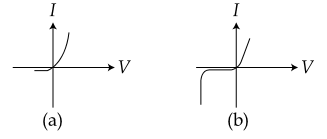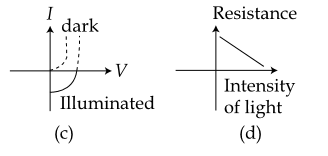#### Identify the semiconductor devices  whose characteristics are given below, in the order (a), (b), (c), (d) :Option 1) Simple diode, Zener diode, Solar cell, Light dependent resistance Option 2) Zener diode, Simple diode, Light dependent resistance, Solar cell Option 3) Solar cell, Light dependent resistance, Zener diode, Simple diod Option 4) Zener diode, Solar cell, Simple diode, Light dependent resistanceAs we learnt in

Zener diode -

It can operate continuously without being damaged in the region of reverse  biased

- wherein

1) It acts as voltage regulator

2) In forward biasing it act as ordinary diode .

Solar cells -

It is based on photovoltic effect .It  convert solar energy into electrical energy .

- wherein

One of the semi conductor region is made so thin that the light incident on it reaches the PN junction and get absorbed .

(a) represents simple diode.

(b) represents zener diode

(c) solar cell

(d) light dependent resistance.

Correct option is 1.

Option 1)

Simple diode, Zener diode, Solar cell, Light dependent resistance

This is the correct option.

Option 2)

Zener diode, Simple diode, Light dependent resistance, Solar cell

This is an incorrect option.

Option 3)

Solar cell, Light dependent resistance, Zener diode, Simple diod

This is an incorrect option.

Option 4)

Zener diode, Solar cell, Simple diode, Light dependent resistance

This is an incorrect option.

#### Plabita